# Electrical characteristics of devices using the IVCharacteristics study object¶

Version: O-2018.06

This tutorial introduces the IVCharacteristics study object. Study objects are special analysis objects, designed to assist the user in setting up and analyzing calculations that require complex computational workflows, and that would otherwise require several individual steps to be performed.## Introduction¶

The IVCharacteristics study object enables the calculation and analysis of the most relevant electrical characteristics of field-effect-transistor (FET) device models, including the on/off ratio ($$\mathrm{I_{on}/I_{off}}$$), the subthreshold slope ($$\mathrm{SS}$$), the transconductance ($$\mathrm{g_{m}}$$) and the drain-induced barrier lowering ($$\mathrm{DIBL}$$) [SK06].

In this tutorial, you will use IVCharacteristics study object to calculate and analyze some of the electrical characteristics of a model silicon-on-insulator (SOI) device, for which experimental results are available for comparison. A preconstructed SOI device will be used, and the IV Characteristics study object will be used to calculate the $$\mathrm{SS}$$, which will be compared with the available experimental results, and the $$\mathrm{DIBL}$$.

## Calculation and preliminary analysis of the $$V_{DG}$$ curve¶

The SOI device model that will be studied is shown in the figure below. it consists of a hydrogen-passivated $$2D$$ silicon slab, two dielectric regions mimicking the $$\mathrm{SiO_2}$$ encapsulation ($$\epsilon = 3.9 \epsilon_0$$), and a metallic gate, positioned at the center of the channel region.For sake of efficiency, the electronic structure of the device model will be calculated using a semi-empirical tight-binding model [BLSJK10].

Download the file containing the DeviceConfiguration and the associated calculator from this link: soi_device.py. Place it in your project directory, and the script will appear in the Project files window of QuantumATK.

Select the file and drag and drop it into the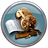Script generator. In theScript generator, change the name of the Results file in the Output settings to ‘soi_device_ivc1.hdf5‘ and add the following analysis block: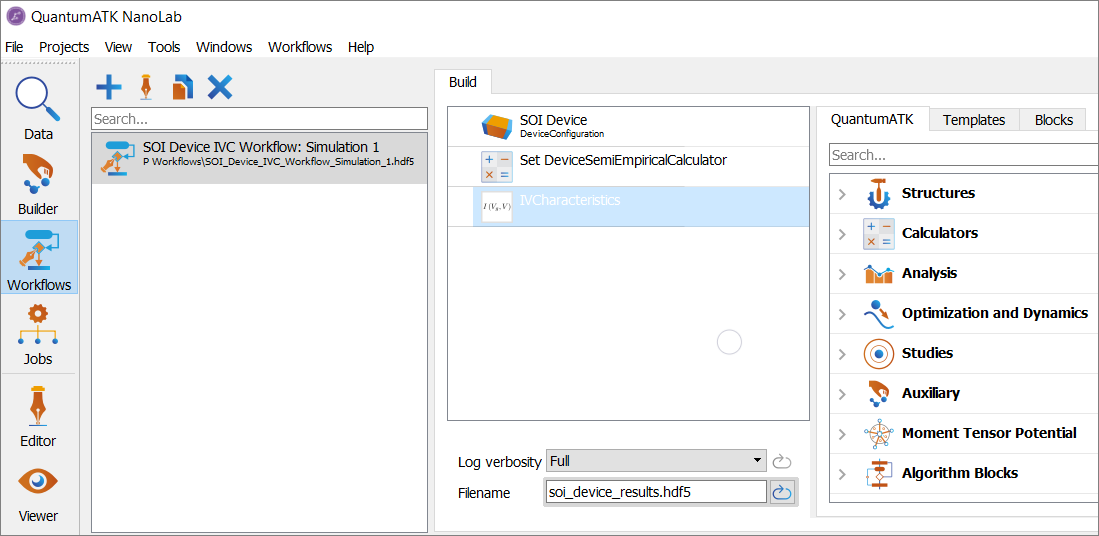Click on the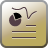IV Characteristics block in order to open it, and modify its parameters as follows:

• In the Gate source voltage range section:

• Set $$\mathrm{V_{gs0}} = -0.3 \mathrm{V}$$.
• Set $$\mathrm{V_{gs1}} = 0.0 \mathrm{V}$$.
• Set number of points 7.
• Tick the option ‘Print results summary to log‘.Note

Using these settings, we have instructed the IVCharacteristics study object to perform a scan of the gate-source bias from $$\mathrm{V_{gs0}} = -0.3 \mathrm{V}$$ to $$\mathrm{V_{gs1}} = 0.0 \mathrm{V}$$, at regular intervals $$\mathrm{\Delta V_{gs}} = 0.05 \mathrm{V}$$ and at a small drain-source bias $$\mathrm{V_{ds}} = 0.05 \mathrm{V}$$.

Once you are done, click OK. In theScripter send the script to the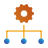Job manager using the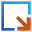button, save it as ‘soi_device_ivc1.py‘ and press thebutton to run the calculation. The calculation will take about 10 minutes on 24 CPUs. You can also download the full script from here: soi_device_ivc1.py.

Once the calculation is done, drag and drop the log file soi_device_ivc1.log into the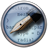Editor. You will notice that a detailed summary of all the planned tasks to be performed by the IVCharacteristics study object has been printed out (here we show only the first lines for briefness):

  1 2 3 4 5 6 7 8 9 10 11 12 13 14 +------------------------------------------------------------------------------+ | IV Characteristics Study | +------------------------------------------------------------------------------+ | 14 task(s) will be executed. | | | | * Update configuration | | Gate voltage: -0.25 V | | Left electrode voltage: 0.05 V | | Right electrode voltage: 0.0 V | | * Calculate TransmissionSpectrum | | Gate voltage: -0.3 V | | Left electrode voltage: 0.05 V | | Right electrode voltage: 0.0 V | | * Update configuration | 

Notice how the complete workflow is shown: a total of 14 different tasks will be executed by the IVCharacteristics study object, and the step-by-step workflow is outlined, with each of the planned tasks marked by an asterisk, and highlighted in yellow in the above figure.

One can also notice that during execution of the workflow, an infobox is printed out for each of the executed tasks:

 1 2 3 4 5 6 7 8 +------------------------------------------------------------------------------+ | Executing task 1 / 14: | | Update configuration | | Gate voltage: 0.0 V | | Left electrode voltage: 0.05 V | | Right electrode voltage: 0.0 V | | Log to: ivcharacteristics_gate_voltage_0.0_Volt.log | +------------------------------------------------------------------------------+ 

Once you are finished inspecting the output, close theEditor and go back to the main QuantumATK window. In the LabFloor, select theivcharacteristics object contained in the file SOI_device_ivc1.hdf5, and click on the IV-Characteristics Analyzer plugin on the right-hand side of the screen.The main panel of the IV-Characteristics Analyzer, shown in the figure below, will appear.On the bottom part of the IV-Characteristics Analyzer panel, a number of additional informations are available:

• Data: information about the convergence of the individual calculations.
• Scale: scale used on the Y axis for the current $$\mathrm{I_{ds}}$$. Can be either Linear or Logarithmic.
• Temperatures: temperature that has been used for the Fermi-Dirac distribution function in the electrodes, when calculating the current $$\mathrm{I_{ds}}$$ using the Landauer formula. The temperature for each electrode can be modified by the user, in which case the currents are recalculated on the fly according to the chosen value.”.

The top part of the IV-Characteristics Analyzer shows a plot of the calculated data, and allows to switch between two different types of visualizations:

• Gate-Source plot: plot of the drain-source current $$\mathrm{I_{ds}}$$ versus the gate-source bias $$\mathrm{V_{gs}}$$ ($$\mathrm{I_{ds}}\ vs. \mathrm{V_{gs}}$$ plot).
• Drain-Source plot: plot of the drain-source current $$\mathrm{I_{ds}}$$ versus the drain-source bias $$\mathrm{V_{ds}}$$ ($$\mathrm{I_{ds}}\ vs. \mathrm{V_{ds}}$$ plot).

Since in the present case only one bias voltage $$\mathrm{V_{ds}}$$ has been considered, only the $$\mathrm{I_{ds}}\ vs. \mathrm{V_{gs}}$$ characteristics will be analyzed.

To calculate the subthreshold slope $$\mathrm{SS}$$, click on the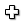button in the Drain-Source Voltage section at the center of the panel and and select the Subthreshold Slope option from the drop-down menu. The range over which the $$\mathrm{SS}$$ is calculated will be highlighted in gray in the $$\mathrm{I_{ds}}\ vs. \mathrm{V_{gs}}$$ plot. You can adjust the Gate voltage range used to calculate the $$\mathrm{SS}$$ in the Subthreshold Slope panel on the right-hand side of the screen.You will immediately notice that the value of $$\mathrm{SS}$$ is very dependent on the chosen Gate voltage range. The reason is that the calculated section of the $$I_{ds}\ vs. V_{gs}$$ curve lies outside the sub-threshold region, where the current $$I_{ds}$$ varies linearly (on a logarithmic scale) with $$V_{gs}$$. Therefore, in order to calculate a more reliable value of the $$\mathrm{SS}$$, the range of $$V_{gs}$$ must be extended to sample correctly the subthreshold region.

## Extending the range of the $$V_{DG}$$ curve¶

In order to sample the sub-threshold region, the range of $$\mathrm{V_{gs}}$$ has to be extended to $$-0.9 \mathrm{V} \leq\ \mathrm{V_{gs}} \leq 0.0 \mathrm{V}$$. This can be achieved in two ways:

### 2A. Extend the range of gate-source voltage in the study object¶

To extend the range in the study object, repeat the operations described in Section 1 to setup the calculation using the IVCharacteristics study object, with the following differences:

• Modify the parameters in the Gate source voltage range section as follows:

• Set $$\mathrm{V_{gs0}} = -0.9 \mathrm{V}$$.
• Set number of points 19.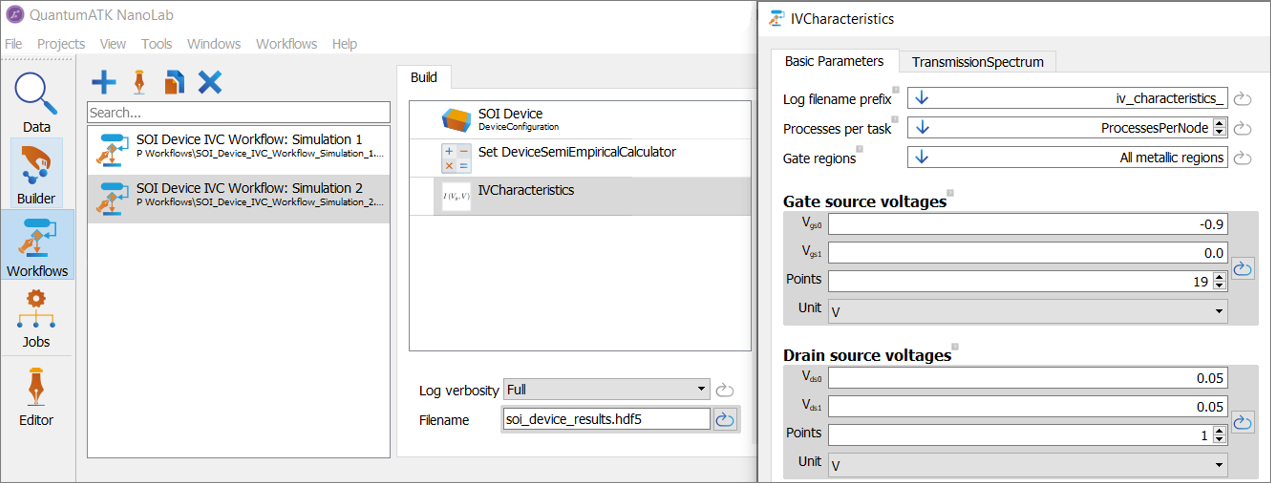Once you are done, click OK. In theScripter, send the script to theJob manager using thebutton, save it as soi_device_ivc2.py and press thebutton to run the calculation. The calculation will take about 15 minutes on 24 CPUs. You can also download the full script from here: soi_device_ivc2a.py.

By inspecting the output file of the calculation, you will notice that only the $$\mathrm{V_{gs}}$$ points which have been not calculated in Section 1 have been calculated. This is due to the fact that the IVCharacteristics study object automatically performs a check of which values of $$\mathrm{V_{gs}}$$ have been already considered, and run calculations only for the remaining values of $$\mathrm{V_{gs}}$$.

Note

Notice how the Gate source voltage range and the number of points have been chosen so that the values of the gate source voltage $$\mathrm{V_{gs}}$$ in the range $$-0.3 \mathrm{V} \leq\ \mathrm{V_{gs}}\ \leq 0.0 \mathrm{V}$$ are consistent with those used in the section Section 1. This ensures that the study object will not repeat any calculation in that gate source voltage range.

Another option to instruct the IVCharacteristics study object to perform calculations for additional values of $$\mathrm{V_{gs}}$$ is to use Python scripting, and add the required voltages by directly modifying the script.

Note

An extensive list of all the command available to interact with the IV Characteristics study object is available in the IVCharacteristics entry of the reference manual.

In order to do this, drag and drop the script soi_device.py in theScripter, and repeat the same operations described in Section 1 to setup the script soi_device_ivc1.py. Also in this case, set the name of the Results file in the Output settings to ‘soi_device_ivc1.hdf5‘.

Once you are done, use thebutton to send the script to theEditor. In theEditor, add the line highlighted in yellow after the IV Characteristics block.

# -------------------------------------------------------------
# Kpoint sampling
kpoint_grid = MonkhorstPackGrid(
na=9,
)

# Gate-source voltages
gate_source_voltages = numpy.linspace(-0.3, 0.0, 7)*Volt

# Drain-source voltages
drain_source_voltages = numpy.linspace(0.05, 0.05, 1)*Volt

# File name.
filename = u'soi_device_ivc1.hdf5'

iv_characteristics = IVCharacteristics(
configuration=device_configuration,
filename=filename,
object_id='ivcharacteristics',
gate_regions=,
gate_source_voltages=gate_source_voltages,
drain_source_voltages=drain_source_voltages,
energies=None,
kpoints=kpoint_grid,
self_energy_calculator=RecursionSelfEnergy(),
energy_zero_parameter=AverageFermiLevel,
infinitesimal=1e-06*eV,
log_filename_prefix='ivcharacteristics_',
)
iv_characteristics.update()


Once you are done, send the script to theJob manager using thebutton, save it as soi_device_ivc2b.py and press thebutton to run the calculation. The calculation will take about 15 minutes on 24 CPUs. You can also download the full script from here: soi_device_ivc2b.py.

## Analysis of the $$V_{DG}$$ curve in the subthreshold region¶

Once the calculations are done, you are ready to analyze the characteristics of the device and extract the subthreshold slope $$\mathrm{SS}$$, this time using the appropriate set of data.

Similarly to what has been already discussed in Section 1, select theivcharacteristics object contained in the file SOI_device_ivc1.hdf5 present in the LabFloor, and click on the IV-Characteristics Analyzer plugin in the right-hand side of the screen.

This time, the IV-Characteristics Analyzer will show an $$\mathrm{I_{DS}}\ vs. \mathrm{V_{GS}}$$ curve, which is perfectly linear in the region of $$-0.9 \mathrm{V} \leq\ \mathrm{V_{GS}}\ \leq -0.35 \mathrm{V}$$. This indicates that this range of $$\mathrm{V_{GS}}$$ corresponds to the subthreshold region, from which it is therefore possible to perform a linear fit to the data and extract the subthreshold slope $$\mathrm{SS}$$.

Click on thebutton in the Drain-Source Voltage section at the center of the panel and select the Subthreshold Slope option from the available options in the drop-down menu. Adjust the Gate voltage range in the Subthreshold Slope panel to include only the subthreshold region by setting it from $$-0.9 \mathrm{V}$$ to $$-0.35 \mathrm{V}$$, as shown in the figure below.The calculated value of the sub-threshold slope is now $$\mathrm{SS} = 123\ \mathrm{meV/dec}$$, in good agreement with the experimentally measured value $$\mathrm{SS}^\mathrm{exp} = 95\ \mathrm{meV/dec}$$ [MMMO12].

## Calculating the drain-induced barrier lowering¶

As a next step, you will calculate the drain-induced barrier lowering ($$\mathrm{DIBL}$$). In order to do so, a second $$\mathrm{I_{DS}}\ vs. \mathrm{V_{GS}}$$ curve will have to be calculated, this time using a higher value of source-drain bias $$\mathrm{V_{DS}}$$, such as $$\mathrm{V_{DS} = 0.3 V}$$.

To setup the new calculation, drag and drop the script soi_device_ivc2b.py to theScripter, add again aStudy Objects ‣ IV Characteristics block, and modify its parameters as follows:

• In the Gate source voltage range section:

• Set $$\mathrm{V_{gs0}} = -0.9 \mathrm{V}$$.
• Set $$\mathrm{V_{gs1}} = 0.0 \mathrm{V}$$.
• Set the number of points to 19.
• In the Drain source voltage range section:

• Set $$\mathrm{V_{ds0}} = 0.05 \mathrm{V}$$.
• Set $$\mathrm{V_{ds1}} = 0.3 \mathrm{V}$$.
• Set the number of points to 2.
• Tick the option ‘Print results summary to log‘.Once you are done, click OK and change the Results file name in the Output settings to ‘soi_device_ivc1.hdf5‘.

Once you are done, send the script to theJob manager using thebutton, save it as soi_device_ivc3.py and press thebutton to run the calculation. The calculation will take about 30 minutes on 24 CPUs. You can also download the full script from here: soi_device_ivc3.py.

Note

By inspecting the log file, you will notice how, thanks to the smart handling of pre-existing calculations, the IV Characteristics object has calculated only the curve at $$\mathrm{V_{DS}} = 0.3 \mathrm{V}$$, using the corresponding points calculated at $$\mathrm{V_{DS}} = 0.05 \mathrm{V}$$ as starting point.

When the calculation is done, select theivcharacteristics object contained in the file SOI_device_ivc1.hdf5 in the LabFloor, and click on the IV-Characteristics Analyzer plugin in the right-hand side of the screen. The IV-Characteristics Analyzer plugin will now show also the two additional curve calculated at $$\mathrm{V_{DS}} = 0.3 \mathrm{V}$$, in addition to that calculated previously at $$\mathrm{V_{DS}} = 0.05 \mathrm{V}$$, as shown in the figure below.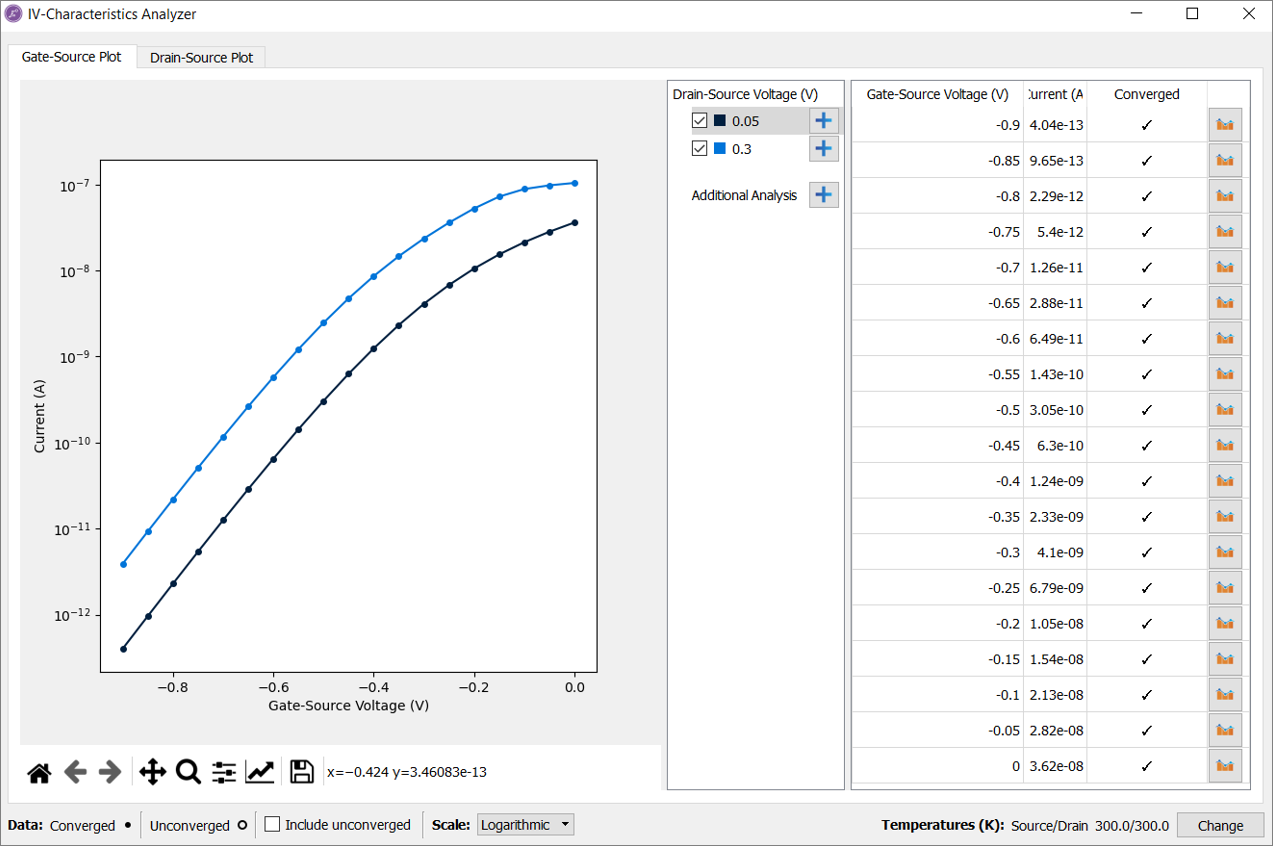To calculate the $$\mathrm{DIBL}$$, click on thebutton next to the Additional Analysis option in the Drain-Source Voltage section at the center of the panel, and select Drain Induced Barrier Lowering from the drop-down menu. The calculated value of $$\mathrm{DIBL}$$ will be shown in the Drain Induced Barrier Lowering section, as shown in the figure below.Note

Notice that the $$\mathrm{DIBL}$$ is given as unitless quantity, therefore the calculated value corresponds to $$\mathrm{622\ mV/V}$$, which is also how the value of the $$\mathrm{DIBL}$$ is most commonly reported.

There are a number of parameters that can be tuned in the Drain Induced Barrier Lowering panel to tune the calculation of the $$\mathrm{DIBL}$$. In particular, it worth mentioning that:

• The Threshold current, which is the value of the current corresponding to a value of $$\mathrm{V_{gs}}$$ equal to the threshold voltage, is set by default at the mid-point between the maximum and the minimum values of the current $$\mathrm{I_{DS}}$$, but can be modified by the user.
• The Subthreshold factor determines the current range used to fit the subthreshold characteristics of the device, in order to determine accurately the threshold voltage. A range containing at least 3 points is requested. If not enough points are included, the $$\mathrm{DIBL}$$ will not be calculated and an error message will be shown instead.
• The Gate voltage range can be modified to include only that part of the curve for which the $$\mathrm{DIBL}$$ should be calculated. This can be important for device models showing bipolar characteristics, where one has two subthreshold regions.

Warning

Note that the calculated value of the $$\mathrm{DIBL}$$ is much larger than that measured experimentally. This can be ascribed to the high sensitivity of the $$\mathrm{DIBL}$$ values to the doping profile and the geometrical characteristics of the device. For ultra-short gate lenghts, values of $$\mathrm{DIBL}$$ between 0.1 and 1 can be expected.